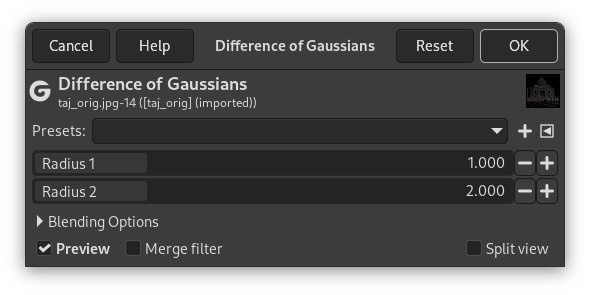### 8.2. Difference of Gaussians

#### 8.2.1. Overview

Figure 17.155.  Applying example for the Difference of Gaussians filterOriginal imageFilter Difference of Gaussians applied with radius 1 = 1.000 and radius 2 = 0.100.

This filter does edge detection using the so-called Difference of Gaussians algorithm, which works by performing two different Gaussian blurs on the image, with a different blurring radius for each, and subtracting them to yield the result. This algorithm is very widely used in artificial vision (maybe in biological vision as well!), and is pretty fast because there are very efficient methods for doing Gaussian blurs. The most important parameters are the blurring radii for the two Gaussian blurs. It is probably easiest to set them using the preview, but it may help to know that increasing the smaller radius tends to give thicker-appearing edges, and decreasing the larger radius tends to increase the threshold for recognizing something as an edge. In most cases you will get nicer results if Radius 2 is smaller than Radius 1, but nothing prevents you from reversing them, and in situations where you have a light figure on the dark background, reversing them may actually improve the result.

#### 8.2.2. Activating the filter

You can find this filter through FiltersEdge-DetectDifference of Gaussians….

#### 8.2.3. Options

Figure 17.156.  Gaussian Difference filter optionsPresets, Preview, Split view
NoteThese options are described in Section 2, “Common Features”.